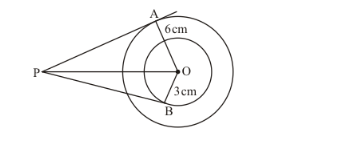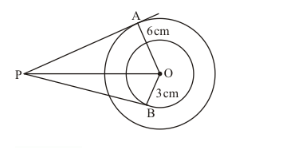# In the given figure, if AP = 10 cm, then BP =

Question:

In the given figure, if AP = 10 cm, then BP =(a) $\sqrt{91} \mathrm{~cm}$

(b) $\sqrt{127} \mathrm{~cm}$

(c) $\sqrt{119} \mathrm{~cm}$

(d) $\sqrt{109} \mathrm{~cm}$

Solution:

Since the radius is always perpendicular to the tangent at the point of contact,$\angle O A P=90^{\circ}$

Therefore,

$O P^{2}=O A^{2}+A P^{2}$

$O P^{2}=6^{2}+10^{2}$

$O P^{2}=36+100$

$O P^{2}=136$

$O P=\sqrt{136}$

Now, consider. Here also, OB is perpendicular to PB since the radius will be perpendicular to the tangent at the point of contact. Therefore,

$P B^{2}=O P^{2}-O B^{2}$

$P B^{2}=(\sqrt{136})^{2}-3^{2}$

$P B^{2}=136-9$

$P B^{2}=127$

$P B=\sqrt{127}$

The correct answer is option (b).